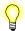# asin(), asinf(), asinl()

Compute the arcsine of an angle

## Synopsis:

```#include <math.h>

double asin( double x );

float asinf( float x );

long double asinl( long double x );```

## Arguments:

x
The sine for which you want to find the angle.

## Library:

libm

Use the -l m option to qcc to link against this library.

## Description:

These functions compute the value of the arcsine (in radians) of x.

## Returns:

The arcsine, in the range (-π/2, π/2). For finite values not in the range [-1,1], these functions return NaN. The return value for +/-Inf is NaN.If an error occurs, these functions return 0, but this is also a valid mathematical result. If you want to check for errors, set errno to 0, call the function, and then check errno again. These functions don't change errno if no errors occurred.

## Examples:

```#include <stdio.h>
#include <math.h>
#include <stdlib.h>

int main( void )
{
printf( "%f\n", asin(.5) );

return EXIT_SUCCESS;
}```

produces the output:

`0.523599`

## Classification:

Safety:
Cancellation point No
Interrupt handler No
Signal handler No